milk>試卷(2021/10/14)

# 110 年 - [無官方正解]110 國立臺灣大學_碩士班招生考試_財務金融研究所乙組:財務管理與財金數學(含微分方程、線性代數)#102120

1.4. (10 points, each question has 2 points) Pleasc write down the right choice for each question.

【題組】(1) Vincent Swift is a risk neutral investor, he will choose
(A)A
(B)B
(C)C
(D)D2.【題組】(2) Here are cash flow forecasts for two mutually exclusive projects.Based on NPV, which project would you choose if the opportunity cost of capital is 12%?
(A) A,
(B) B,
(C)the same,
(D)cannot be decided.

3.【題組】(3) Based on M&M model, A firm has a debt-to-equity ratio of 1.0. If it had no debt, its cost of equity would be 15%. Its cost of debt is 10%. What is its cost of equity if there are no taxes or other imperfections?
(A)21%.
(B)18%.
(C)15%.
(D)20%.

4.【題組】(4) Both call and put options are impacted by the ex-dividend date. Put options become more and Call options become________ due to the dividend payment.
(A) expensive, expensive
(B)cheaper, cheaper
(C)expensive, cheaper
(D)cheaper, expensive.

5.【題組】(5) A company (NTU Ltd) that has paid a dividend of \$4 this year. Assuming a higher growth for the next 3 years at 12% and stable growth of 6% thereafter, let's calculate the value of the stock using a two-stage dividend discount model. The required rate of return is 10%.
(A)4.48
(B)5.95
(C)124.33
(D)154.54

【非選題】
6.
1. (15 points) Capital structure overlooks that firms have different capital requirements across their life cycle. In addition, the finance literature highlights various theoretical models that explain how the firms' life stages influence capital struc cture decisions, such as the Pecking Order, Trade-Off, and Agency Cost Theories. We like to know how firms in highly innovative industries have' different capital requirements over their life cycle.

【題組】(1) (5points) In the beginning of the life cycle, firms are more likely to raise funds through issuing equity or using borrowing, why?

【非選題】
7.【題組】(2) (5 points) When firms are matured, they are more likely to use debt or equity financing, why?

【非選題】
8.【題組】(3) (5 points) Tony Lin is a founder of a highly innovative company. The company is facing a high-growth period. Tony insists to use debt financing to support the firm's new investment. Does Tony make the right decision? Why, or why not? Why does Tony make such a decision? Is there anything related to his personal preference?

【非選題】
9.

2. (15 points) To construct a portfolio using the mean-variance framework, one needs cstimates of the expected return r, the variance  σ2i and the co-variance aj for each stocks, j. Forn stocks, you have to estimate a total of n(n-1)/2 correlation coefficients. Single index models are used, to reduce this hugc amount of needed estimates. Now we are going to construct a portfolio with two assets using the single index model.

【題組】

(1) (3 points) Please calculate the mean and variance of the stock index return and mean return for asset B.We run the following regression for each asset.【非選題】
10.【題組】

(2) (2 points) Please find out the estimates of αi and βi for Asset A【非選題】
11.【題組】

(3) (4 points) A Single Index Model (SIM) specifies two sources of uncertainty for a security's 21.078 return: Systematic risk and firm-specific risk Return variance Systematic risk Firm-specific risk【非選題】
12.【題組】

(4) (6 points) Now assuming a portfolio with weights for asset A and asset B are 0.7 and 0.3. Plcase find out E(rp) and【非選題】
13.
3.(10 points) The equity in a firm is a residual claim, i.e., equity holders lay claim to all cashflows left over after other financial claim-holders (debt, preferred stock ctc,) have been satisfied. Ifa firm is liquidated, the same principle applies, with equity investors receiving whatever is left over in the firm atter all outstanding debts and other financial claims are paid off. The principle of limited liability, however, protects equity investors in publicly traded firms if the value of the firm is less than the valuc of the outstanding debt, and they cannot lose more than their investment in the firm.

【題組】(1) (2 points) Beside the residual claim right mentioned above, what elise rights that an equity investor hold?

【非選題】
14.【題組】

(2) (1.5 point) The payoff to equity investors, on liquidation, can therefore be written as: Payofwhere, V = Value of the firm
D = Facc Value of the outstanding debt and other external
Please draw a figure of the payoff to equity investors (Y-axis) and the firr value (X-axis).

【非選題】
15.【題組】

(3) (1.5 point) The payoff to equity investors, on liquidation, can therefore be written as: Payoffwhere, V = Value of the firm
D = Face Value of the outstanding debt and other external
Piease draw a figure of the payoff to debt investors (Y-axis) and the firm value (X-axis)

【非選題】
16.【題組】

(4) The relationship between equity/debt and firm value can be described by the following assets, call option, put option, and pure bond. Please fill in the correct position (long, short, or NO) for an investor to take to desctribe the relation between equity/debt and firm value. (3 points)Please explain tour reasons. (2 points) Put option

【非選題】
17.

1. (5 points) Consider the system of following questionswhere x1, x2, x3 are unknown. Please use the matrix-vector product to express the 3 x 3 linear system

【非選題】
18.2. (5 points) Following previous question, please find reduced row cchelon form (iret)

【非選題】
19.

3. (5 points) Please find a matrix C solution so that (BC)T - A = 0, where【非選題】
20.

4. Consider a two-period economy (/ = 0, 1). The operating profit of firm j can be expressed as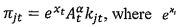is the exogenous profitability shock; At is a technology shook at time t;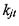is private capital of fimm j. a ＞ 0 is assumed. The depreciation rate of capital k for firm / is δj. Thus, the investment of firm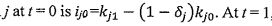, firm j has a liquidation value of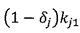. The investment in private capital involves adjustment cost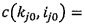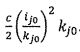. The stochastic discount factor between time 0 and 1 is m, where log(m) = logβ + γ(x0 -x1), where 0 ＜β＜ 1, and γ＞ 0. Firm j then chooses ij.0 to maximize its value, which is the sum of the discounted cash flows from the two periods: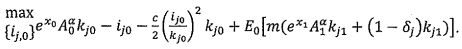E[.] is an expectation operator. From standard asset pricing equation,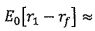-Cov0(r1,m). Specifically, the cash flows from t = 0 are equal to the operating profit minus the investment cost and the adjustment cost. The cash flows from ! = I are equal to the operating profit plus the liquidation value.

【題組】(1) (10 points) Please drive FOC for maximum firm value, and express it as a form of marginal cost equal to marginal revenue.

【非選題】
21.【題組】

(2) (10 points) Note that the investment return, defined as the ratio of marginal benefit to marginal cost of investment, is equal to the firm's stock return (rl) under equilibrium. Please show that【非選題】
22.【題組】

(3) (10 points) Please derive expected excess return:, which is a function of the covariance between the operating profit at t = 1 of firm y and the aggregate productivity shock.

【非選題】
23.【題組】(4) 5 points) Show that the expected excess return of firm j is positively associated with technology shock at time 1.

### 懸賞詳解

#### 國一地理下第三次

40.（ ）在臺灣區域計畫的劃分中，目前哪兩個區域因工商業發展程度高，導致環境汙染、擁擠問題較嚴重？　(A)北部、中部　(B)中部、東部　(C)南部、...